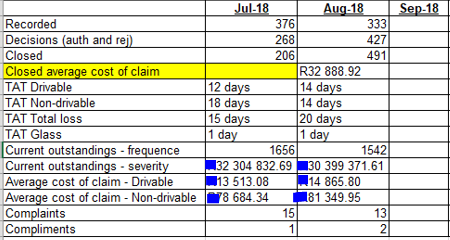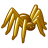# QlikView App Dev

Discussion Board for collaboration related to QlikView App Development.

Announcements
Join this live chat April 6, 10AM EST - QlikView to Qlik Sense REGISTER
cancel
Showing results for
Search instead for
Did you mean:Creator II

## Pivot or Straight table

Hi Champs

I need to create report like below:Details:

I have to type Recorded, Decisions,close,TAT Glass etc. manually. but I do have formulas on how to calculate each details per Month

E.g. Recorded= Count(RecDate) and Current Outstanding-frequence(count(open claims))

The problem is how to display it this way in qlikview?

I cant attach data cause it is big and  has sensitive info.

Thanks

1 Solution

Accepted SolutionsPartner

try this

1-

Sum({<Month = {">=\$(=Month(YearStart(Today())))<=\$(=Month(Today()))"}>} total sales)

2-

1- Sum({<BU = {'MX'}>} Sales)

2-Sum({<BU = {'Ooba'}>} Sales)

Thanks

Raman

4 RepliesPartner

try this approach

Make a pivot table with Month as dimension and Recored,Decision,close .. etc yours expressions and change your dimension position in pivot table .

Regards

Raman RastogiCreator II
Author

Thanks Raman

Quick Questions

1) I need to do set analysis for total to start from Jan to current month like below Above  pic?

2) Is two graphs same calculation but the first graph pulls data where item BU is =MX and second is  where item BU is =Ooba please assist with correct syntax please.

ThanksMVP

This way may give proper result, If format is good

 1) I need to do set analysis for total to start from Jan to current month like below Above  pic?

Sum({<Month = {">=\$(=Month(YearStart(Today())))<=\$(=Month(Today()))"}>} TOTAL Sales)

 2) Is two graphs same calculation but the first graph pulls data where item BU is =MX and second is  where item BU is =Ooba please assist with correct syntax please.

2.1) Sum({<BU = {'MX'}>} Sales)

2.2) Sum({<BU = {'Ooba'}>} Sales)

Before develop something, think If placed (The Right information | To the right people | At the Right time | In the Right place | With the Right context)Partner

try this

1-

Sum({<Month = {">=\$(=Month(YearStart(Today())))<=\$(=Month(Today()))"}>} total sales)

2-

1- Sum({<BU = {'MX'}>} Sales)

2-Sum({<BU = {'Ooba'}>} Sales)

Thanks

Raman